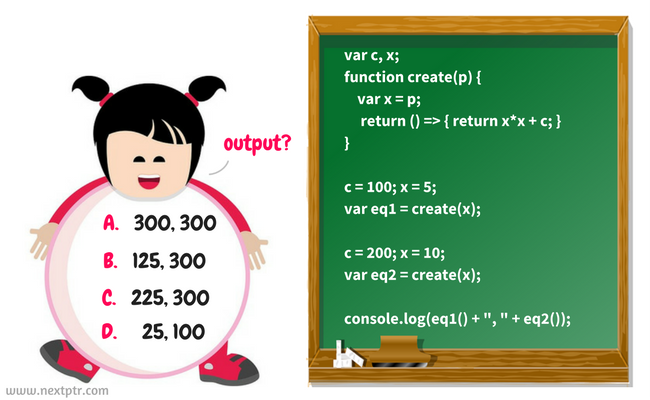# What would be the output of calling these two closure functions?The create function returns a closure function that captures a global variable c and a local variable x in parent scope. The returned closure is an equation `x^2+c`. We modify global variables and call create twice to get 2 closures, eq1 and eq2. You have to tell what would be the output of calling these 2 closures:

``````var c, x; // global

// returns closure
function create(p) {
var x = p;
return () => { return x*x + c; }
}

// change c, x and create eq1
c = 100; x = 5;
var eq1 = create(x);

// change c, x and create eq2
c = 200; x = 10;
var eq2 = create(x);

// call both eq1 and eq2
console.log(eq1() + ", " + eq2());
``````

What would be the output?